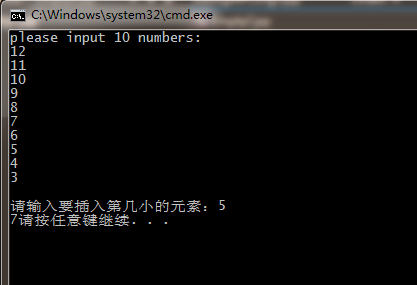C++ 寻找第K小元素（不排序）

``````#include <iostream>
using namespace std;
int searchk(char a, int k)
{
int num = 0;
for (int i = 0; i < 10; i++)
{
num = a[i];
int temp = 0;
for (int j = 0; j < 10; j++)
{
if (num > a[j])
temp++;
}
if (temp == k - 1)
return num;
}
}
int main()
{
char a;
int k;
cout << "please input 10 numbers:";
for (int i = 0; i < 10; i++)
{
cin >> a[i];
}
cout << endl << "请输入要插入第几小的元素：";
cin >> k;
searchk(a, k);
system("pause");
}
``````

2个回答

``````#include <iostream>
using namespace std;

int searchk(int a, int k)
{
int result; //返回结果
int numberOfLess; //假如要找第3小的数，numberOfLess= 2，以此类推
for (int i = 0; i < 10; i++)
{
result = a[i];

numberOfLess = 0;
for (int j = 0; j < 10; j++)
{

if (j != i) //无需判断自身
{
if (a[j] < a[i])
{
numberOfLess++; //记录比a[i]小的数
}
}

}

if (numberOfLess == k-1)//当找到第k个小的数时，跳出整个循环
{
break;
}

}
return result; //返回找到的数
}
int main()
{
int a;
int k;
cout << "please input 10 numbers:";
for (int i = 0; i < 10; i++)
{
cin >> a[i];
}
cout << endl << "请输入要插入第几小的元素：\n";
cin >> k;
int outPut = searchk(a,k);
cout << outPut;
system("pause");
}
``````jalen_zh 回复CYW_N: 真的！像你这个问题，肯定少不了排序啊，而且你的数据没有什么特殊的地方。无论怎样写，都是需要排序
3 年多之前 回复CYW_N 谢谢~真的吗？那么要怎样的形式才算不排序啊？
3 年多之前 回复